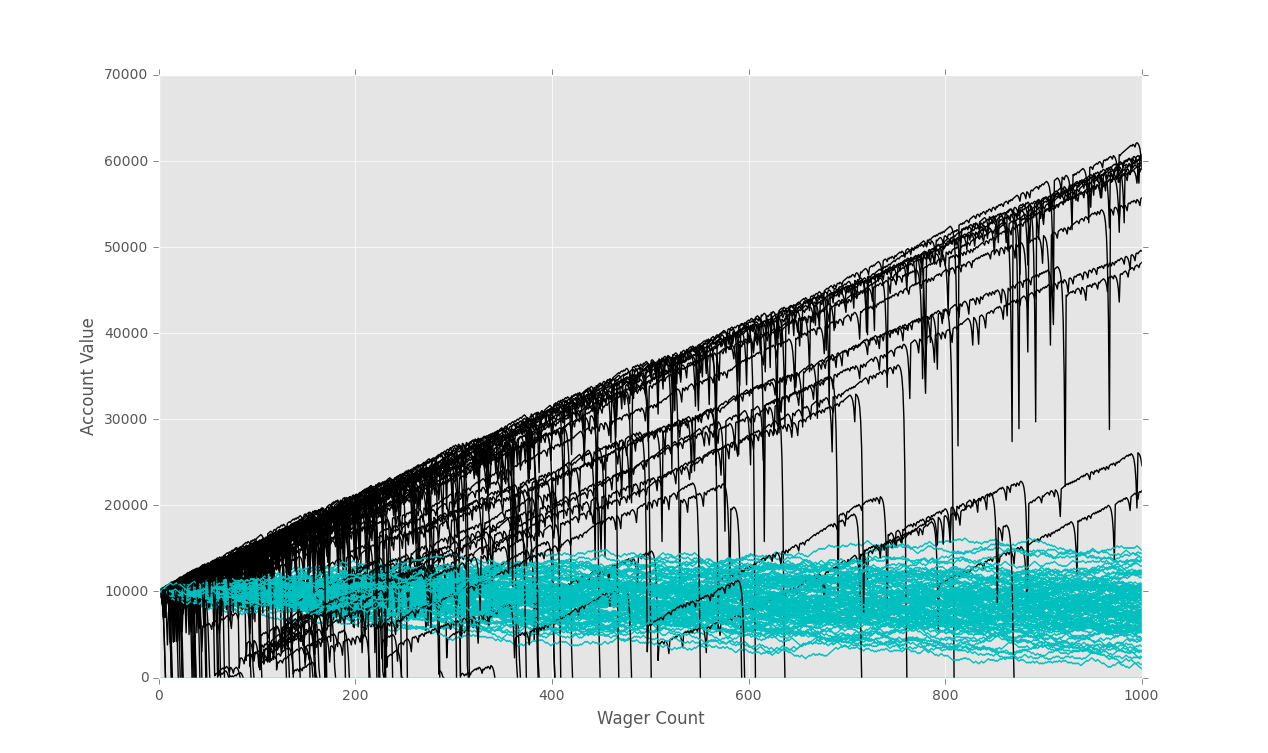## Fixing Debt Issues

So, now that we've got this far, we've found that our bettors can go under 0.

For the \$100 bettor, this is not the biggest deal, but still might allow for many bettors to get much further.

For the doubler bettor, this can prove to be "life changing" so to speak.

Let's take away the ability for our bettors to get into the negatives next, so we can fairly compare them, since our 100 dollar bettor can only go into negative 100, but the doubler bettor can go into the negative hundreds of thousands. This isn't fair.

```import random
import matplotlib
import matplotlib.pyplot as plt
import time

sampleSize = 100

startingFunds = 10000
wagerSize = 100
wagerCount = 1000

def rollDice():
roll = random.randint(1,100)

if roll == 100:
return False
elif roll <= 50:
return False
elif 100 > roll >= 50:
return True

################################################## edit in color
def doubler_bettor(funds,initial_wager,wager_count,color):

value = funds
wager = initial_wager
wX = []
vY = []
currentWager = 1
previousWager = 'win'
previousWagerAmount = initial_wager

while currentWager <= wager_count:
if previousWager == 'win':
if rollDice():
value += wager
wX.append(currentWager)
vY.append(value)
else:
value -= wager
previousWager = 'loss'
previousWagerAmount = wager
wX.append(currentWager)
vY.append(value)
if value < 0:
currentWager += 10000000000000000
elif previousWager == 'loss':
if rollDice():
wager = previousWagerAmount * 2

# this makes it so we just bet all that is left.
if (value - wager) < 0:
wager = value

value += wager
wager = initial_wager
previousWager = 'win'
wX.append(currentWager)
vY.append(value)
else:
wager = previousWagerAmount * 2
# this makes it so we just bet all that is left.
if (value - wager) < 0:
wager = value
value -= wager
previousWager = 'loss'
previousWagerAmount = wager
wX.append(currentWager)
vY.append(value)

# change to equals zero!
if value <= 0:
currentWager += 10000000000000000

currentWager += 1
########################### this guy edits color #
plt.plot(wX,vY,color)

'''
Simple bettor, betting the same amount each time.
'''
#####                                           color#
def simple_bettor(funds,initial_wager,wager_count,color):

value = funds
wager = initial_wager
wX = []
vY = []
currentWager = 1
while currentWager <= wager_count:
if rollDice():
value += wager
wX.append(currentWager)
vY.append(value)
else:
value -= wager
wX.append(currentWager)
vY.append(value)

### change this part, not lessthan or equal zero, it is zero
if value <= 0:
currentWager += 10000000000000000
currentWager += 1

# this guy goes green #
plt.plot(wX,vY,color)

x = 0

while x < sampleSize:
simple_bettor(startingFunds,wagerSize,wagerCount,'c')
#simple_bettor(startingFunds,wagerSize*2,wagerCount,'c')
doubler_bettor(startingFunds,wagerSize,wagerCount,'k')
x+=1

plt.axhline(0, color = 'r')
plt.ylabel('Account Value')
plt.xlabel('Wager Count')
plt.show()
```The next tutorial:• Monte Carlo Introduction

• Monte Carlo dice Function

• Creating a simple Bettor

• Plotting Results with Matpltolib

• Martingale Strategy

• Bettor Statistics

• More comparison

• Graphing Monte Carlo

• Fixing Debt Issues
• Analyzing Monte Carlo results

• Using Monte Carlo to find Best multiple

• Checking betting results

• D'Alembert Strategy

• 50/50 Odds

• Analysis of D'Alembert

• Comparing Profitability

• Finding best D'Alembert Multiple

• Two dimensional charting monte carlo

• Monte Carlo Simulation and Python

• Labouchere System for Gambling Tested